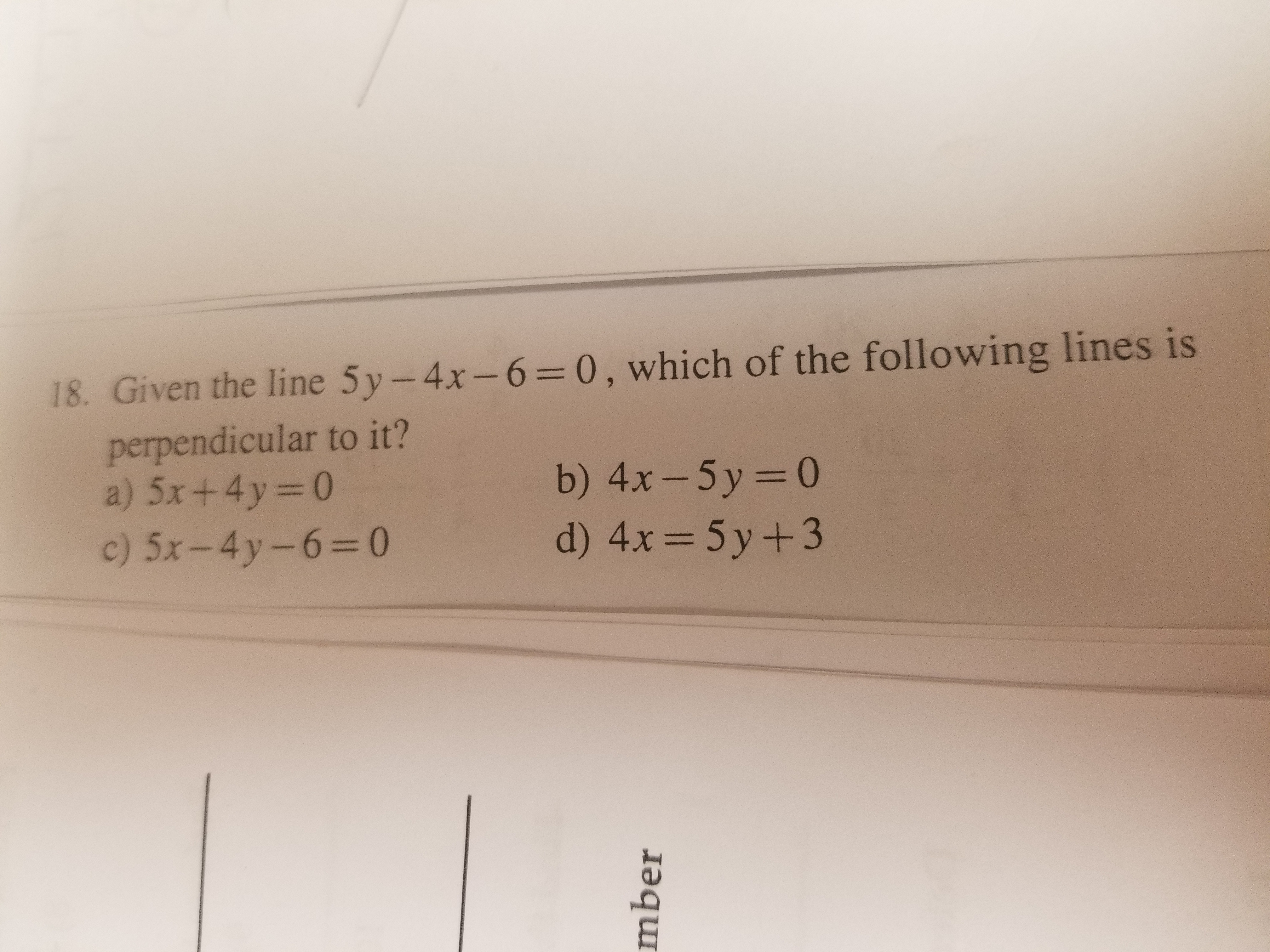# 18. Given the line 5y-4x-60, which of the following lines isperpendicular to it?a) 5x + 4y = 0b) 4x-5y=0d) 4x 5y +32

Question
6 viewshelp_outlineImage Transcriptionclose18. Given the line 5y-4x-6 0, which of the following lines is perpendicular to it? a) 5x + 4y = 0 b) 4x-5y=0 d) 4x 5y +3 2 fullscreen
check_circle

Step 1

Given information:

The given equation of line is 5y-4x-6=0

Procedure Used:

Step 1: Write the given equation of line in slope intercept form.

Step 2: Evaluate the slope of the given line.

Step 3: Using the below relation, evaluate the slope of the perpendicular line.

Step 4: Compare the above slope with all the slope of line.

Step 2

Calculation :

First, write the given equation of line in slope intercept form.

Step 3

Compare the above equation with the equation y=mx+b, we get

m=4/5  ...

### Want to see the full answer?

See Solution

#### Want to see this answer and more?

Solutions are written by subject experts who are available 24/7. Questions are typically answered within 1 hour.*

See Solution
*Response times may vary by subject and question.
Tagged in

### Equations and In-equations